Next: Born Approximation Up: Scattering Theory Previous: Introduction

# Fundamental Equations

Consider time-independent scattering theory, for which the Hamiltonian of the system is written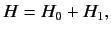(910)

whereis the Hamiltonian of a free particle of mass,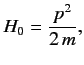(911)

and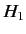represents the non-time-varying source of the scattering. Let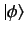be an energy eigenket of,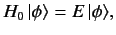(912)

whose wavefunction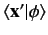is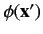. This state is assumed to be a plane wave state or, possibly, a spherical wave state. Schrödinger's equation for the scattering problem is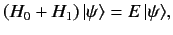(913)

where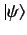is an energy eigenstate of the total Hamiltonian whose wavefunction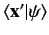is. In general, bothand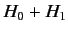have continuous energy spectra: i.e., their energy eigenstates are unbound. We require a solution of Equation (913) that satisfies the boundary condition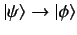as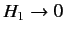. Here,is a solution of the free particle Schrödinger equation, (912), corresponding to the same energy eigenvalue.

Adopting the Schrödinger representation, we can write the scattering problem (913) in the form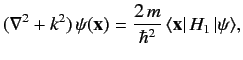(914)

where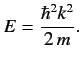(915)

Equation (914) is called the Helmholtz equation, and can be inverted using standard Green's function techniques. Thus,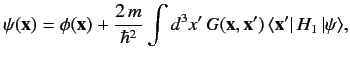(916)

where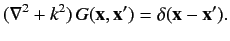(917)

Note that the solution (916) satisfies the boundary conditionas. As is well-known, the Green's function for the Helmholtz problem is given by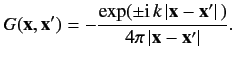(918)

Thus, Equation (916) becomes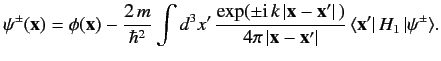(919)

Let us suppose that the scattering Hamiltonian,, is only a function of the position operators. This implies that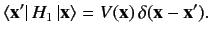(920)

We can write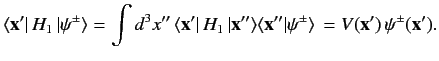(921)

Thus, the integral equation (919) simplifies to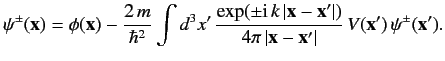(922)

Suppose that the initial stateis a plane wave with wavevector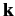(i.e., a stream of particles of definite momentum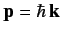). The ket corresponding to this state is denoted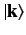. The associated wavefunction takes the form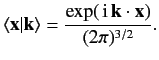(923)

The wavefunction is normalized such that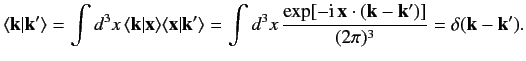(924)

Suppose that the scattering potentialis only non-zero in some relatively localized region centered on the origin (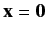). Let us calculate the wavefunction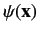a long way from the scattering region. In other words, let us adopt the ordering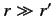. It is easily demonstrated that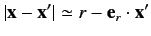(925)

to first order in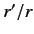, where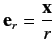(926)

is a unit vector that points from the scattering region to the observation point. Here,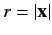and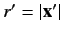. Let us define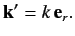(927)

Clearly,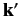is the wavevector for particles that possess the same energy as the incoming particles (i.e.,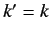), but propagate from the scattering region to the observation point. Note that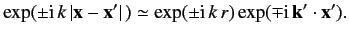(928)

In the large-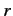limit, Equation (922) reduces to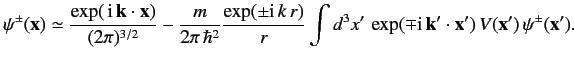(929)

The first term on the right-hand side is the incident wave. The second term represents a spherical wave centred on the scattering region. The plus sign (on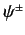) corresponds to a wave propagating away from the scattering region, whereas the minus sign corresponds to a wave propagating towards the scattering region. It is obvious that the former represents the physical solution. Thus, the wavefunction a long way from the scattering region can be written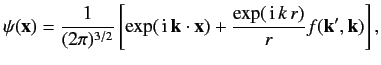(930)

where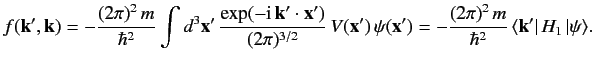(931)

Let us define the differential cross-section,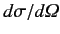, as the number of particles per unit time scattered into an element of solid angle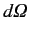, divided by the incident flux of particles. Recall, from Chapter 3, that the probability current (i.e., the particle flux) associated with a wavefunctionis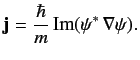(932)

Thus, the probability flux associated with the incident wavefunction,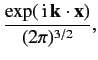(933)

is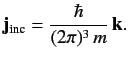(934)

Likewise, the probability flux associated with the scattered wavefunction,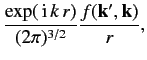(935)

is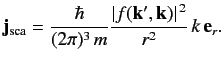(936)

Now,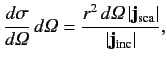(937)

giving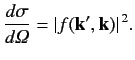(938)

Thus,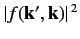gives the differential cross-section for particles with incident momentum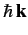to be scattered into states whose momentum vectors are directed in a range of solid anglesabout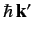. Note that the scattered particles possess the same energy as the incoming particles (i.e.,). This is always the case for scattering Hamiltonians of the form specified in Equation (920).Next: Born Approximation Up: Scattering Theory Previous: Introduction
Richard Fitzpatrick 2013-04-08